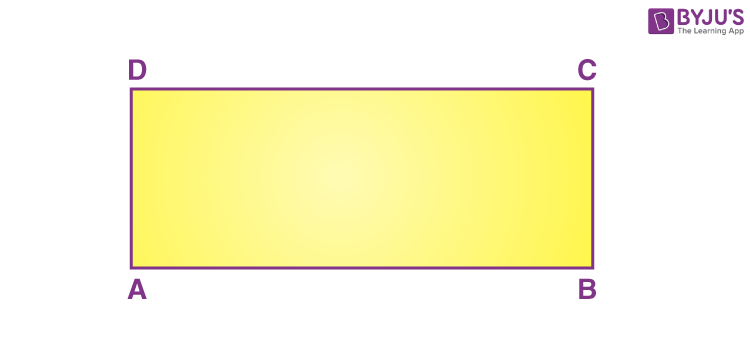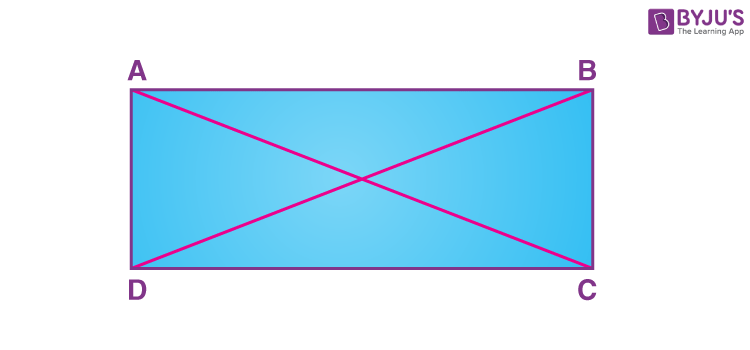# Rectangle

A Rectangle is a four sided-polygon, having all the internal angles equal to 90 degrees. The two sides at each corner or vertex, meet at right angles. The opposite sides of the rectangle are equal in length which makes it different from a square.

For example, if one side of a rectangle is 20 cm, then the side opposite to it is also 20 cm.A rectangle is characterized by length (L) and width (W). Both length and width are different in size.

In the figure above, a rectangle ABCD has four sides as AB, BC, CD, and DA and right angles A, B, C, and D. The distance between A and B or C and D is defined as the length (L), whereas the distance between B and C or A and D is defined as Width (W) of the given rectangle.

## Definition

A rectangle is a type of quadrilateral that has its parallel sides equal to each other and all the four vertices are equal to 90 degrees. Hence, it is also called an equiangular quadrilateral.

Since, the opposite sides are equal and parallel, in rectangle, therefore, it can also be termed as a parallelogram.

## Shape of Rectangle

A rectangle is a two-dimensional flat shape. In an XY plane, we can easily represent a rectangle, where the arms of x-axis and y-axis show the length and width of the rectangle, respectively.

### Rectangular Shaped Objects

The most common everyday things or objects we see and are rectangular in shape is Television, computer screen, notebook, mobile phones, CPU , Notice boards, Table, Book, TV screen, Mobile phone, Wall, Magazine, Tennis court, etc.

## Properties of Rectangle

The properties of rectangle are given below:

 It has four sides and four vertices Each vertex has angle equal to 90 degrees The opposite sides are equal and parallel Diagonal bisect each other Perimeter is equal to twice of sum of its length and breadth Area is equal to product of its length and breadth It’s a parallelogram with four right angles. Sum of all interior angles equal to 360 degrees

## Perimeter of a Rectangle

The perimeter of a rectangle is defined as the total distance covered by the outer boundary of the rectangle. It is measured in unit length. The formula of perimeter is given by:

Perimeter, P = 2 (Length + Width)

## Area of Rectangle

Area is the region covered by a two-dimensional shape in a plane. It is measured in square units. Therefore, the area of the rectangle is the area covered by its outer boundaries. It is equal to the product of length and width.

The formula of area of rectangle is:

$$\begin{array}{l}A = Length \times Width \;\; unit^{2}\end{array}$$

## Diagonal of a Rectangle

A rectangle has two diagonals, that bisects each other. Both the diagonals are equal in length.### Length of Diagonals

The rectangle is a symmetrical shape and has both the diagonals equal in length. A diagonal will divide the rectangle into two right angle triangles. Therefore we can easily calculate the length of diagonals using the Pythagoras Theorem, where the diagonals are considered as hypotenuse of the right triangle.

Let D is the hypotenuse, length (L)  and width (W) are the base and perpendicular, respectively. Hence, the length of the diagonal of rectangle will be:

$$\begin{array}{l}D = \sqrt{L^{2}+W^{2}}\end{array}$$

## Video Lesson on Properties of rectangles### Solved Example

 Example- Find the Area and Perimeter of a rectangle where length and width are given as 12 and 8 cm respectively. Also, find the length of the Diagonal. Solution- We know that the area of a rectangle is given by $$\begin{array}{l}A = Length \times Width\end{array}$$. $$\begin{array}{l}\Rightarrow A = 12 \times 8\end{array}$$ $$\begin{array}{l}\Rightarrow A = 96 cm^{2}\end{array}$$ Now Perimeter is given by $$\begin{array}{l}P = 2 (Length + Width) \end{array}$$ $$\begin{array}{l}\Rightarrow P = 2 (12 + 8) \end{array}$$ $$\begin{array}{l}\Rightarrow P = 40 \end{array}$$ Diagonal Length, $$\begin{array}{l}D = \sqrt{L^{2}+W^{2}}\end{array}$$ $$\begin{array}{l}\Rightarrow D = \sqrt{12^{2}+8^{2}}\end{array}$$ $$\begin{array}{l}\Rightarrow D = \sqrt{144 + 64} \end{array}$$ $$\begin{array}{l}\Rightarrow D = \sqrt{208} \end{array}$$ $$\begin{array}{l}\Rightarrow D = 4\sqrt{13}\end{array}$$

Register at BYJU’S to learn more properties of different shapes and figures in a fun and creative way.

## Frequently Asked Questions – FAQs

Q1

### What is a rectangle in Geometry?

A rectangle is a type of quadrilateral, whose opposite sides are equal and parallel. It is a four-sided polygon that has four angles, equal to 90 degrees. A rectangle is a two-dimensional shape.
Q2

### Is square a rectangle?

A square can be considered a rectangle based on few properties: opposite sides are equal and parallel, all angles are equal to 90 degrees, diagonals bisect each other, sum of all angles equal to 360 degrees.
Q3

### What is the shape of rectangle?

A rectangle is closed flat shape, having four sides, and each angle equal to 90 degrees. The opposite sides of the rectangle are equal and parallel.
Q4

### How to find the area of a rectangle?

Area of rectangle is equal to the product of its length and width. Suppose, length of rectangle is 5cm and width is 4 cm, then the area will be:
Area = 5cm x 4cm = 20 sq.cm.
Q5

### What is the rectangle formula?

The rectangle formulas are:
Area = L x B
Perimeter = 2 (L+B)
Diagonal = √(L2+B2)
 Learn more on Rectangles Surface Area Of Rectangle Special parallelograms :Rhombus, Square, Rectangle